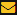﻿ IJLRET IJLRET provides individual hard copy of certificates to all authors after online publication. w.e.f. 01/11/2015
 Log in || Registereditor@ijlret.com

# Current Issue [Vol. 08, No. 09] [September 2022]

 Paper Title :: On the High Dimensional RSA Algorithm--- A Public Key Cryptosystem Based on Lattice and Algebraic Number Theory Author Name :: Zhiyong Zhenga || Fengxia Liub || Man Chen Country :: China Page Number :: 01-16
The most known of public key cryptosystem was introduced in 1978 by Rivest, Shamir and Adleman and now called the RSA public key cryptosystem in their honor. Later, a few authors gave a simply extension of RSA over algebraic numbers field( see -), but they require that the ring of algebraic integers is Euclidean ring, this requirement is much more stronger than the class number one condition. In this paper, we introduce a high dimensional form of RSA by making use of the ring of algebraic integers of an algebraic number field and the lattice theory. We give an attainable algorithm (see Algorithm I below) of which is significant both from the theoretical and practical point of view. Our main purpose in this paper is to show that the high dimensional RSA is a lattice based on public key cryptosystem indeed, of which would be considered as a new number in the family of post-quantum cryptography(see  and ). On the other hand, we give a matrix expression for any algebraic number fields (see Theorem 2.7 below), which is a new result even in the sense of classical algebraic number theory
Key Words: RSA, The Ring of Algebraic Integers, Ideal Matrix, Ideal Lattice, HNF Basis
. M. Ajtai, C. Dwork. A Public-Key Cryptosystem with Worst-Case/Avarage -Case Equivalence. 29th ACM Symposium on Theory of Computing, 1997, 284-293.
. D. Bonech. Twenty Years of Attacks on the RSA Cryptosystem. Notices of the Ams, 2002, 46(2): 203-213.
. D. Coppersmith. Finding Small Solutions to Small Degree polynomials. Lecture Notes in Computer Science, 2001, 2146: 20-31.
. H. Cohen. A Course in Computational Algebraic Number Theory, Graduate Texts in Mathematics. Springer-Verlag, 1993.
. P. J. Davis. Circulant Matrices. 2nd Edition, Chelseea Publishing, New York, 1994.

 Copyright © 2015 IJLRET. All Right Reseverd Home   Editorial Board   Current Issue   Contact Us